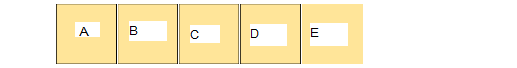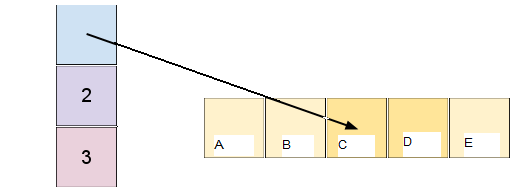## Array

``````var astring
a = "A"
a = "B"
a = "C"
a = "D"
a = "E"
// array 只要宣告了變數即可使用，不需要做初始化
``````## Slice

``````var a[]int
a = make([]int,5,5)
a = "A"
a = "B"
a = "C"
a = "D"
a = "E"
// slice 需要使用 make 做初始化才能開始使用
````````````s = a[2:4]
//s == "C"
//s == "D"
//len(s) == 2
//cap(s) == 3
s = "Z"
// a == "Z" 這段是拿來證明 s是參照到 a
``````Slice 切片出來的東西，都是創建一個新的Slice指向原本的Slice，所以原本的abcde slice，被切成 2-4，就會變成只有 C 跟 D, 但他的容量(cap)一開始規劃出來是 5，5-2=3，長度(len)則是 4-2=2。

`Slice 有一個重點就是在於 長度(len)不可大於(cap)否則會噴錯。`

## Slice 陷阱

`copy 是 buit-in function`

``````//做一個跟 a 長度相同的 slice
c:=make([]string,len(a))
//這樣子 c 就是複製出來的 Slice 而不是參考指向
copy(c,s)
``````